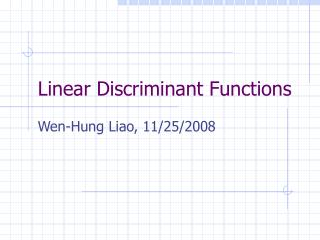Download PresentationLinear Discriminant FunctionsLinear Discriminant Functions - PowerPoint PPT Presentation

Download PresentationLinear Discriminant Functions
An Image/Link below is provided (as is) to download presentation

Download Policy: Content on the Website is provided to you AS IS for your information and personal use and may not be sold / licensed / shared on other websites without getting consent from its author. While downloading, if for some reason you are not able to download a presentation, the publisher may have deleted the file from their server.

- - - - - - - - - - - - - - - - - - - - - - - - - - - E N D - - - - - - - - - - - - - - - - - - - - - - - - - - -
Presentation Transcript

1. Linear Discriminant Functions Wen-Hung Liao, 11/25/2008

2. Introduction: LDF • Assume we know the proper form of the discriminant functions, instead of the underlying probability densities. • Use samples to estimate the parameters of the classifier.(statistical or non-statistical) • Will be concerned with discriminant functions that are either linear in the components of x, or linear in some given set of functions of x.

3. Why LDF? • Simplicity vs. accuracy • Attractive candidates for initial, trial classifiers • Related to neural networks

4. Approach • Find the LDF by minimizing a criterion function. • Use gradient descent procedure for minimization • Convergence property • Computational complexities • Example of criterion function: Sample risk, or training error. (Not appropriate, why?) Because a small training error does not guarantee a small test error.

5. LDF and Decision Surfaces • A linear discriminant function: where w : weight vector w0: bias or threshold

6. Two-Category Case • Decision rule: • Decide w1 if g(x) > 0, decide w2 if g(x)<0 • In other words, x is assigned to w1 if the inner product wtx exceeds the threshold –w0.

7. Decision Boundary • A hyperplane H defined by g(x)=0 • If x1 and x2 are both on the decision surface, then: • w is normal to any vector lying on the hyperplane.

8. Distance Measure • For any x, where xp is the normal projection of x onto H , and r is the algebraic distance.

9. Multi-category Case • General case: • c-1 2-class c(c-1)/2 linear discriminant

10. Use c linear discriminants

11. Distance Measure • wi-wj is normal to Hij. • Distance for x to Hij is given by:

12. Quadratic DF • Add terms involving products of pairs of component of x to obtain the quadratic discriminant function: • The separating surface defined by g(x)=0 is a hyperquadric function.

13. Hyperquadric Surfaces • If W=[wij] is not singular, then the linear terms in g(x) can be eliminated by translating the axes. • Define a scale matrix: • Hypersphere • Hyperellipsoid • Hyperperboloid

14. Generalized LDF • Polynomial discriminant functions • Generalized LDF:

15. Augment Vectors • Augment feature vector: • Augment weight vector: • Mapping a d-dimensional x-space to (d+1)-dimensional y-space

16. 2-Category Separable Case • Look for a weight vector that classifies all of the samples correctly. If such a weight does exist, then the samples are said to be linearly separable.

17. Gradient Descent Procedure • Define a criterion function J(a) that is minimized if a is a solution vector. • Step 1: Randomly pick a(1), and compute the gradient vector: • Step 2: a(2) is obtained by moving some distance from a(1) in the direction of the steepest descent.

18. Setting the Learning Rate • Second-order expansion of J(a): • Substituting • Minimized when

19. Newton Descent • For nonsingular H • Converges faster but more difficult to compute per step.

20. Perceptron Criterion Function where Y(a) is the set of samples misclassified by a. • Since • Update rule:

21. Convergence Proof • Refer to page 229 to 232 of textbook.